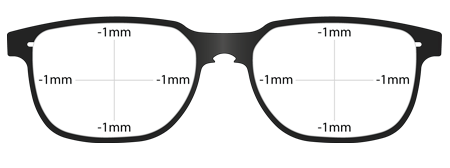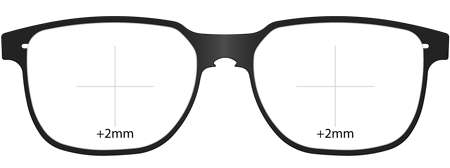# SPD Sizes

Staffan Preutz Design can be tailored to fit perfectly for the best cosmetic and optical effect. The size modifications available are listed below:

• *1 = -2mm each lens
• *2 = -4mm each lens
• *3 = +2mm each lens
• *4 = +4mm each lens
• *5 = -2mm in width each lens
• *6 = -4mm in width each lens
• *7 = +2mm in width each lens
• *8 = +4mm in width each lens
• *9 = +2mm on bottom of each lensTo reduce each lens size by 2mm in width and height as above, order size change *1 (-2mm).To add 2mm in depth to the shape, useful for accommodating progressive lenses order size change *9 (+2mm on bottom).

NOTE: Distance between lenses (DBL) remains constant when changing size.